Next: The Kinetic Monte Carlo Up: Introduction Previous: Introduction   Contents

## Graph Theory Representation of a Finite-state Markov Chain

In general, to fully define a Markov chain it is necessary to specify all the possible states of the system and the rules for transitions between them. Graph theoretical representations of finite-state Markov chains are widely used [198,187,199,200]. Here we adopt a digraph [154,201] representation of a Markov chain, where nodes represent the states and edges represent the transitions of non-zero probability. The edge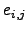describes a transition from node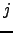to node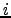and has a probability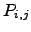associated with it, which is commonly known as a routing or branching probability.A node can be connected to any number of other nodes. Two nodes of a graph are adjacent if there is an edge between them .

For digraphs all connections of a node are classified as incoming or outgoing. The total number of incoming connections is the in-degree of a node, while the total number of outgoing connections is the out-degree. In a symmetric digraph the in-degree and out-degree are the same for every node .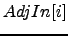is the set of indices of all nodes that are connected to nodevia incoming edges that finish at node. Similarly,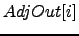is the set of the indices of all the nodes that are connected to nodevia outgoing edges from node. The degree of a graph is the maximum degree of all of its nodes. The expectation value for the degree of an undirected graph is the average number of connections per node.

For any nodethe transition probabilities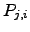add up to unity,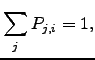(6.1)

where the sum is over all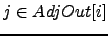. Unless specified otherwise all sums are taken over the set of indices of adjacent nodes or, since the branching probability is zero for non-adjacent nodes, over the set of all the nodes.

In a computer program dense graphs are usually stored in the form of adjacency matrices . For sparse graphs  a more compact but less efficient adjacency-lists-based data structure exists . To store a graph representation of a Markov chain, in addition to connectivity information (available from the adjacency matrix), the branching probabilities must be stored. Hence for dense graphs the most convenient approach is to store a transition probability matrix  with transition probabilities for non-existent edges set to zero. For sparse graphs, both the adjacency list and a list of corresponding branching probabilities must be stored.Next: The Kinetic Monte Carlo Up: Introduction Previous: Introduction   Contents
Semen A Trygubenko 2006-04-10